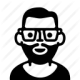# Top 17 VLSI Interview Questions & Answers

1) Explain how logical gates are controlled by Boolean logic?

In Boolean algebra, the true state is denoted by the number one, referred as logic one or logic high. While, the false state is represented by the number zero, called logic zero or logic low.  And in the digital electronic, the logic high is denoted by the presence of a voltage potential.

2) Mention what are the different gates where Boolean logic are applicable?

• NOT Gate: It has one out input and one output. For example, if the value of A= 0 then the Value of B=1 and vice versa
• AND Gate: It has one output due to the combination of two output. For example, if the value of A and B= 1 then value of Q should be 1
• OR Gate: Either of the value will show the same output. For example, if the value of A is 1 or B is 0 then value of Q is 1

These are the basic three types of gates where Boolean logic work, apart from these, other gates that are functional works with the combination of these three basic gates, they are XNOR gate, NAND gate, Nor gate and XOR gate.

3) Explain how binary number can give a signal or convert into a digital signal?

Binary number consists of either 0 or 1, in simple words number 1 represents the ON state and number 0 represents OFF state. These binary numbers can combine billion of machines into one machines or circuit and operate those machines by performing arithmetic calculations and sorting operations.

4) Mention what is the difference between the TTL chips and CMOS chips?

 TTL Chips CMOS Chips TTL chips for transistor transistor logic. It uses two Bi-polar Junction Transistors in the design of each logic gate TTL chips can consist of a substantial number of parts like resistors TTLS chip consumes lot more power especially at rest. A single gate in TTL chip consumes about mW of power TTL chips can be used in computers CMOS stands for Complementary Metal Oxide Semi-conductor. It is also an integrated chip but used field effect transistors in the design CMOS has greater density for logic gates. In a CMOS chip, single logic gate can comprise of as little as two FETs CMOS chips consume less power. A single CMOS chip consume about 10nW of power CMOS chip is used in Mobile phones

5) Explain what is a sequential circuit?

A sequential circuit is a circuit which is created by logic gates such that the required logic at the output depends not only on the current input logic conditions, but also on the sequences past inputs and outputs.6) Explain how Verilog is different to normal programming language?

Verilog can be different to normal programming language in following aspects

• Simulation time concept
• Basic circuit concepts like primitive gates and network connections

7) Explain what is Verilog?

Verilog is an HDL (Hardware Description Language) for describing electronic circuits and systems.  In Verilog, circuit components are prepared inside a Module.  It contains both behavioral and structural statements.  Structural statements signify circuit components like logic gates, counters and micro-processors. Behavioral statements represent programming aspects like loops, if-then statements and stimulus vectors.

8) In Verilog code what does “timescale 1 ns/ 1 ps” signifies?

In Verilog code, the unit of time is 1 ns and the accuracy/precision will be upto 1ps.

9) Mention what are the two types of procedural blocks in Verilog?

The two types of procedural blocks in Verilog are

• Initial: Initial blocks runs only once at time zero
• Always: This block loop to execute over and again and executes always, as the name suggests

10) Explain why present VLSI circuits use MOSFETs instead of BJTs?

In comparison to BJT, MOSFETS can be made very compact as they occupy very small silicon area on IC chip and also in term of manufacturing they are relatively simple. Moreover, digital and memory ICs can be employed with circuits that use only MOSFETs, i.e., diodes, resistors, etc.

11) Mention what are three regions of operation of MOSFET and how are they used?

MOSFET has three regions of operations

• Cut-off region
• Triode region
• Saturation region

The triode and cut-off region are used to function as a switch, while, saturation region is used to operate as an amplifier.

12) Explain what is the depletion region?

When positive voltage is transmitted across Gate, it causes the free holes (positive charge) to be pushed back or repelled from the region of the substrate under the Gate. When these holes are pushed down the substrate, they leave behind a carrier depletion region.

13) Explain why is the number of gate inputs to CMOS gates usually limited to four?

Higher the number of stacks, slower the gate will be.  In NOR and NAND gates the number of gates present in the stack is usually alike as the number of inputs plus one. So input are restricted to four.

14) Explain what is multiplexer?

A multiplexer is a combination circuit which selects one of the many input signals and direct to the only output.

15) Explain what is SCR (Silicon Controlled Rectifier)?

SCR is a 4 layered solid state device which controls current flow.  It is a type of rectifier that is controlled by a logical gate signal. It is a 4 layered, 3-terminal device.

16) Explain what is Slack?

Slack is referred as a time delay difference from the expected delay to the actual delay in a particular path. Slack can be negative or positive.

17) Explain what is the use of defpararm?

With the keyword defparam, parameter values can be configured in any module instance in the design.

1.